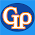## Friday, January 2, 2015

### Expansion of gases and Applications

Similar to the liquids gases also has no specific area and length and hence there are no linear and areal expansions for a gas. We need not worry about the apparent expansion and real expansion in the case of the gases. It is simply because the volume expansion of the gases so large such that it is not going to make a difference whether it is apparent expansion or real expansion.

While studying the expansion of solids and liquids we need to worry about the impact of pressure. But pressure plays important role during the expansion or the contraction of the gases and we cannot ignore the parameter. Studying the variation of the volume both with respect to temperature and pressure simultaneously leads to unwanted confusion. That’s why we will be keeping one of the parameter constant and steady the other two parameters.

Hence gases have two types of expansion coefficients. They are volume expansion coefficient of a gas at constant pressure and pressure expansion coefficient of a gas at constant volume.

Volume expansion coefficient of a gas at constant pressure is defined as the ratio of change in the volume of the gas to its original volume at 0°C per 1°C rise in temperature at constant pressure.

Pressure expansion coefficient of a gas at constant volume is defined as the ratio of change in the pressure to its original pressure at 0°C per 1° c rise in temperature at constant volume.

We can find the relation between these two coefficients as shown below. It is noticeable that both are identical. Irrespective of any of the gas, the expansion coefficients are same.

When a graph is drawn between the volume and temperature at the constant pressure, it can be noticed that with the increase of the temperature volume of the gas also increases. With the decrease of the temperature volume starts decreasing and at a temperature -273°C volume of any gas becomes zero. This particular temperature is called as absolute zero temperature. This is taken like a reference to design a scale and the scale is called as absolute scale or Kelvin scale.

Boyle’s law

At constant temperature, for a given mass of the gas pressure is inversely proportional to volume.

Boyle’s law is not treated like a fundamental law because it is true only under certain conditions like mass has to be constant.

Charles law

At constant pressure, volume of the gases directly proportional to absolute temperature and vice versa.

A gas which satisfies all gas laws at all temperatures and pressures is called an ideal gas. No gas in real life is ideal. All the existing gases are called real gases, which obey gas laws only at high temperatures and low pressures.

We can derive the equation for the ideal gas equation as shown below.

While verifying the Boyle’s law we can verify has the product of pressure and volume equal to constant. When the gas is taken in a uniform tube of certain cross-section we can write the volume of the gases the product of area and length. As the area is constant we can verify the Boyle’s law by proving that the product of pressure and length is also constant.

Problem and solution

If the pressure of a gas is increased by 10% at constant temperature what happens to its volume ?

Related Posts

1.2.